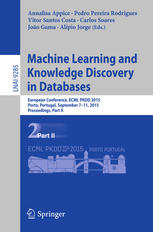• A
• A
• A
• ABC
• ABC
• ABC
• А
• А
• А
• А
• А
Regular version of the site

## Fast Generation of Best Interval Patterns for Nonmonotonic Constraints

P. 157-172.

In pattern mining, the main challenge is the exponential explosion of the set of patterns. Typically, to solve this problem, a constraint for pattern selection is introduced. One of the first constraints proposed in pattern mining is support (frequency) of a pattern in a dataset. Frequency is an anti-monotonic function, i.e., given an infrequent pattern, all its superpatterns are not frequent. However, many other constraints for pattern selection are not (anti-)monotonic, which makes it difficult to generate patterns satisfying these constraints. In this paper we introduce the notion of projection-antimonotonicity and theta-SOFIA algorithm that allows efficient generation of the best patterns for some nonmonotonic constraints. In this paper we consider stability and Δ-measure, which are nonmonotonic constraints, and apply them to interval tuple datasets. In the experiments, we compute best interval tuple patterns w.r.t. these measures and show the advantage of our approach over postfiltering approaches.

### In bookVol. 9285. Prt. 2. Cham; Heidelberg; NY; Dordrecht; L.: Springer, 2015.